# Colleges with the lowest SAT scores in North Carolina

Top 10 colleges in North Carolina with the lowest SAT scores
Looking for the colleges with the lowest SAT scores in North Carolina? Well you're in luck! We've compiled a national college database and have created a list of the top 10 universities with the lowest SAT scores in North Carolina below. If you are not a good test taker or worried about your test scores, this list is for you. These are the schools whose applicants had the lowest average SAT scores in North Carolina, which means that you can get into these colleges with a lower SAT score. We also include each college's ACT scores and acceptance rate so that you can see where you would have the easiest time getting in. Read on to find out more.

## Shaw University SAT scores

The average SAT score for Shaw University is 851.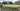The average SAT score of 851 breaks down into:

• SAT math: 420

The average ACT score for Shaw University is 15 and their acceptance rate is 52.5%.

## Chowan University SAT scores

The average SAT score for Chowan University is 910.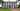The average SAT score of 910 breaks down into:

• SAT math: 440

The average ACT score for Chowan University is 16 and their acceptance rate is 54.7%.

## North Carolina Wesleyan College SAT scores

The average SAT score for North Carolina Wesleyan College is 970.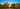The average SAT score of 970 breaks down into:

• SAT math: 480

The average ACT score for North Carolina Wesleyan College is 19 and their acceptance rate is 97.8%.

## Winston-Salem State University SAT scores

The average SAT score for Winston-Salem State University is 970.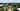The average SAT score of 970 breaks down into:

• SAT math: 490

The average ACT score for Winston-Salem State University is 17 and their acceptance rate is 54%.

## Elizabeth City State University SAT scores

The average SAT score for Elizabeth City State University is 971.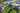The average SAT score of 971 breaks down into:

• SAT math: 480

The average ACT score for Elizabeth City State University is 18 and their acceptance rate is 59.4%.

## North Carolina Central University SAT scores

The average SAT score for North Carolina Central University is 980.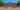The average SAT score of 980 breaks down into:

• SAT math: 490

The average ACT score for North Carolina Central University is 18 and their acceptance rate is 38.2%.

## University of North Carolina Pembroke SAT scores

The average SAT score for University of North Carolina Pembroke is 1001.The average SAT score of 1001 breaks down into:

• SAT math: 500

The average ACT score for University of North Carolina Pembroke is 20 and their acceptance rate is 81.2%.

## Saint Andrews University SAT scores

The average SAT score for Saint Andrews University is 1010.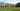The average SAT score of 1010 breaks down into:

• SAT math: 510

The average ACT score for Saint Andrews University is 19 and their acceptance rate is 41.1%.

## Lees McRae College SAT scores

The average SAT score for Lees McRae College is 1030.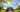The average SAT score of 1030 breaks down into:

• SAT math: 510

The average ACT score for Lees McRae College is 20 and their acceptance rate is 57.2%.

## The University of Mount Olive SAT scores

The average SAT score for The University of Mount Olive is 1065.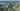The average SAT score of 1065 breaks down into:

• SAT math: 510